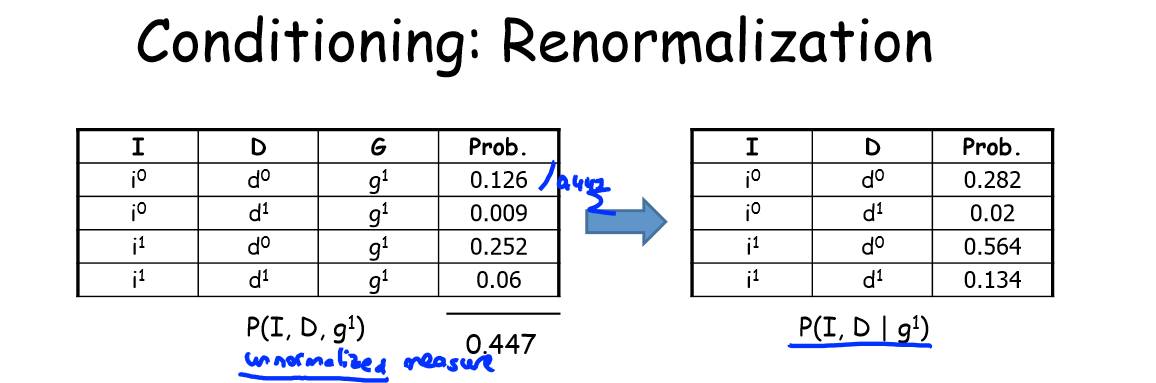## 条件概率分布、联合概率分布与边缘概率分布

1.联合概率分布

①离散型联合概率分布：X和Y的联合概率分布可以表示为：X的概率分布如下：
P（X=0）=0.4
P（X=1）=0.6

Y的概率分布如下：
P（Y=0）=0.25
P（Y=1）=0.5
P（Y=2）=0.25

P(X=0，Y=0)=0.1
P(X=0，Y=1)=0.2
P(X=0，Y=2)=0.1
P(X=1，Y=0)=0.15
P(X=1，Y=1)=0.3
P(X=1，Y=2)=0.15

②连续型联合概率分布：X和Y的联合概率分布可以表示为：2.条件概率分布

P（X=xi|Y=yi）=P（X=xi，Y=yi）/P（Y=yi）3.边缘概率分布

P（I=0）=0.126+0.168+0.126+0.009+0.045+0.126
P（I=1）=0.252+0.0224+0.0056+0.06+0.036+0.024